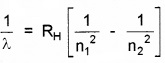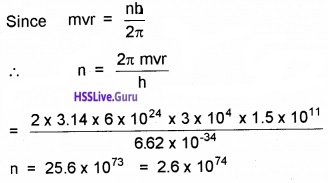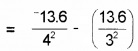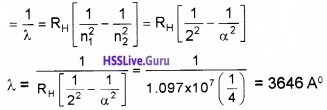# Plus Two Physics Chapter Wise Questions and Answers Chapter 12 Atoms

Students can Download Chapter 12 Atoms Questions and Answers, Plus Two Physics Chapter Wise Questions and Answers helps you to revise the complete Kerala State Syllabus and score more marks in your examinations.

## Kerala Plus Two Physics Chapter Wise Questions and Answers Chapter 12 Atoms

### Plus Two Physics Atoms NCERT Text Book Questions and Answers

Question 1.
What is the shortest wavelength present in the Paschen series of spectral lines?
SinceFor Paschen series, n1 = 3 and n2 = ∞Question 2.
The radius of the innermost electron orbit of a hydrogen atom is 5.3 × 10-11 m. What are the radii of the n = 2 and m = 3 orbits?
Given r1 = 5.3 × 10-11m
n1 = 1, n2 = 2, r2 = ?
n3 = 3, r3 = ?
Since r ∝ n2
∴ r1 ∝ n12
And r2 ∝ n22or r2 = 4 × r1 = 4 × 5.3 × 10-11
or e2 = 2.12 × 10-10m
Also r3 ∝ n32or r3 = 9r1 = 9 × 5.3 × 10-11
or r3 = 4.77 × 10-10m.Question 3.
A 12.5 eV electron beam is used to bombard gaseous hydrogen at room temperature. What series of wavelength will be emitted?
First excitation energy
E = E2 – E1 = -3.4 – (-13.6) = 10.2 eV
Second excitation energy
E = E3 – E1 = -1.51 -(-13.6) = 12.09 eV
Third excitation energy
E = E4 – E1 = -0.85 – (-13.6) = 12.75 eV
Second incident beam has energy = 12.5 eV
So only first two lines in the Lyman series of wave-length 103 nm and 122 nm will be emitted.
Also E3 – E2 = -1.51 – (-3.4) = 4.91 eV
i.e, first line in the Balmer series of wavelength 656nm will also be emitted.Question 4.
In accordance with the Bohr’s model, find the quantum number that characterises the earth’s revolution around the sun in an orbit of radius 1.5 × 1011 m with orbital speed 3 × 104 ms-1.
Here r= 1.5 × 1011 m,
v = 3 × 104 ms-1, m = 6 × 1024kg, n = ?### Plus Two Physics Atoms One Mark Questions and Answers

Question 1.
A radioactive nucleus emits beta particle. The parent and daughter nuclei are
(a) isotopes
(b) isotones
(c) isomers
(d) isobars
(d) isobarsQuestion 2.
Consider an electron in the nth orbit of a hydrogen atom in the Bohr model. The circumference of the orbit can be expressed in terms of de Broglie wavelength λ of that electron as
(a) (0.529) nλ
(b) $$\sqrt{n} \lambda$$
(c) (13.6)λ
(d) nλ
(d) nλ

Question 3.
The ionization energy of hydrogen atom is 13.6 eV. Find the energy corresponding to a transition between 3rd and 4th orbit.
E = E4 – E3= -0.85 + 1.51 = 0.66 eV.Question 4.
If 13.6 eV energy is required to ionize the hydrogen atom, then the energy required to remove an electron from n = 2 is
(a) 10.2 eV
(b) 0 eV
(c) 3.4 eV
(d) 6.8 eV
(c) 3.4 eV
Explanation:= 3.4 eV.

Question 5.
In the Bohr model of the hydrogen atom, the lowest orbit corresponds to
(a) infinite energy
(b) maximum energy
(c) minimum energy
(d) zero energy
(c) minimum energy.Question 6.
Write down the Balmerformula for wavelength of Ha line.### Plus Two Physics Atoms Two Mark Questions and Answers

Question 1.
Given Rydberg constant as 1.097 × 10-7m-1. Find the longest and shortest wavelength limit of Baler Series.
$$\bar{v}=\frac{1}{\lambda}=R_{H}\left[\frac{1}{n_{1}^{2}}-\frac{1}{n_{2}^{2}}\right]$$
Longest wavelength n1 = 2 and n2 = 3Shortest Wavelength n1 = 2 and n2 = α### Plus Two Physics Atoms Three Mark Questions and Answers

Question 1.
Bohr combined classical and early quantum concept and gave his theory in the form of three postulates.

1. The total energy of an electron in ground state of hydrogen atom is -13.6eV. What is the significance of negative sign?
2. The radius of innermost electron orbit of hydrogen atom is 5.3 × 1011m. What are the radii of n = 2 and n = 3 orbits?